XRobotix Assignment 1
Arduino - Piece of Cake
NAME
Question 1 ARDUINO
What is Arduino? Choose all the right options.
Question 2 ARDUINO BOARD
Name components 1 to 6 (Figure 1 below)
Figure 1 Arduino Board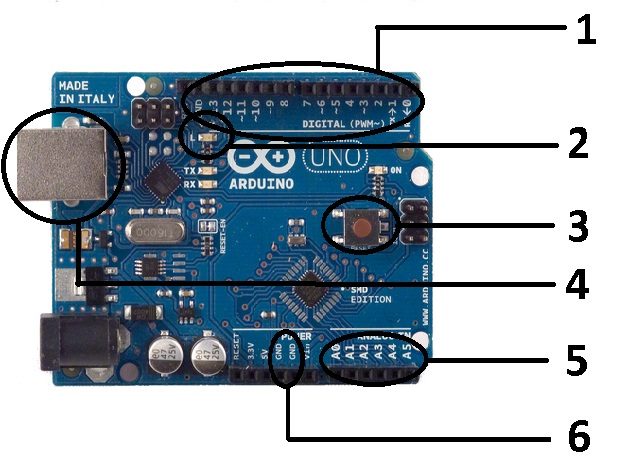Question 3 OUTPUT
What type of wave is represented in Figure 2?
Figure 2 WAVE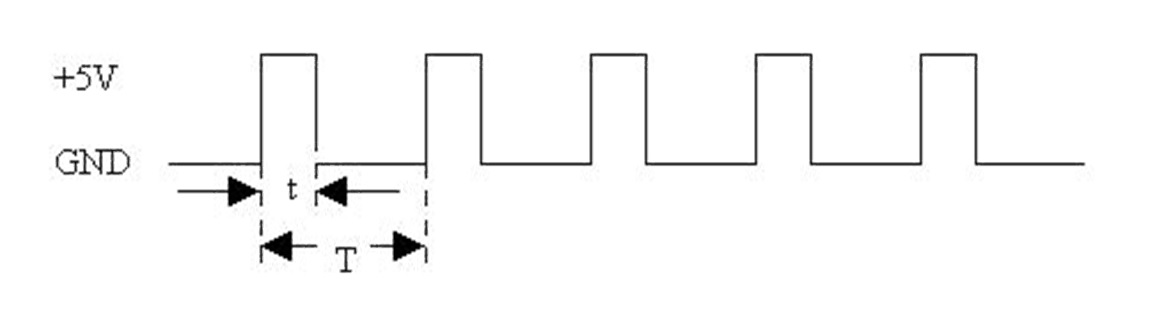Question 4 PROGRAMMING
What is the difference between int and char? Demonstrate with an example.
Question 5 ARDUINO PROGRAMMING
Show how you would set up a basic Arduino program with the build-in LED ON for 2 seconds and then OFF.
Write a Blink Function. (Tip: only use loop)
What is wrong with the connection in Figure 3?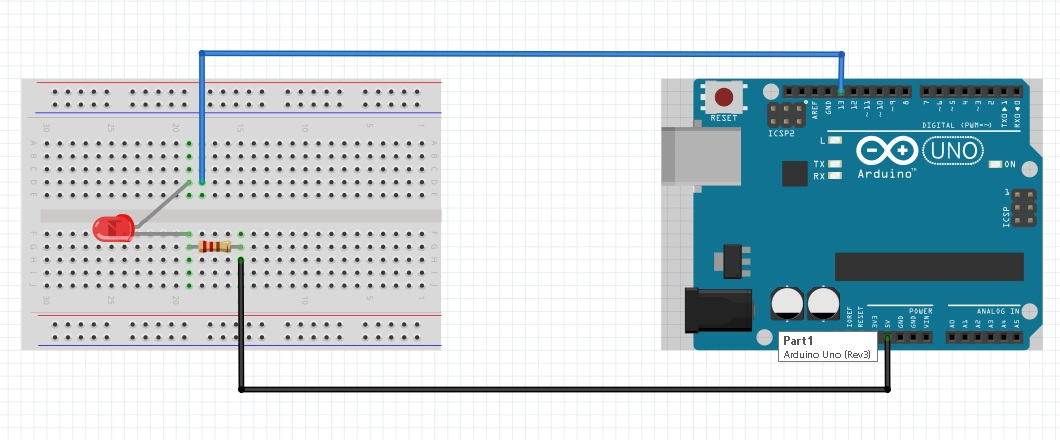Question 8 RESISTORS
A 22kOhm resistor would make a light dimmer/brighter than a 1kOhm resistor connected to a 5V battery.
Question 9 LED'S
Write the void setup() function for 2 LED's.
Question 14a RGB LED
In the case of a common cathode RGB LED, name (+/-) the pins (marked 1-4) in Figure 6 below? (Also name the colours of each pin)
Figure 6 RGB LED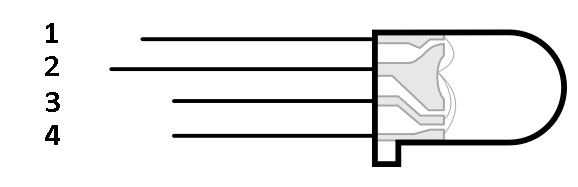Question 14b RGB CONNECTION
To which pins on the Arduino would you connect 1 to 5 in Figure 7 in the case of a common cathode RGB LED?
Figure 7 RGB Connection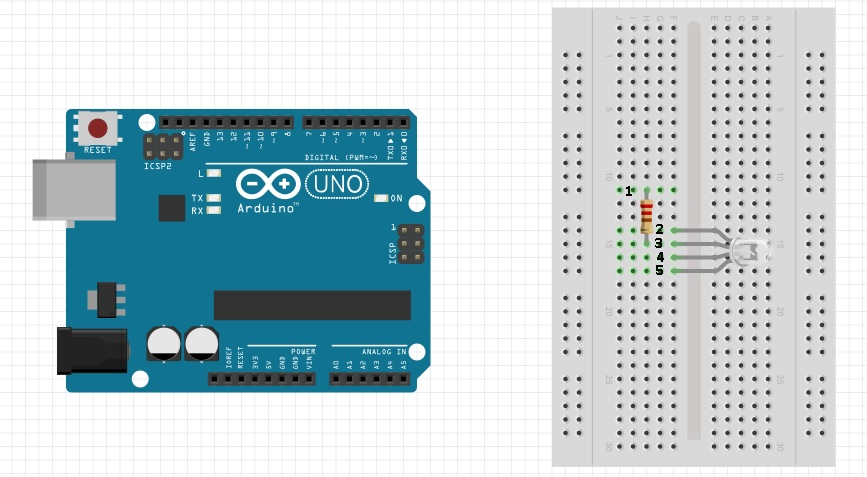Question 14c RGB PROGRAMMING
How would you write a RGB function that the red light is on for one second and then the blue light? Use the setup() function.
asdf *
sdddddd
Required